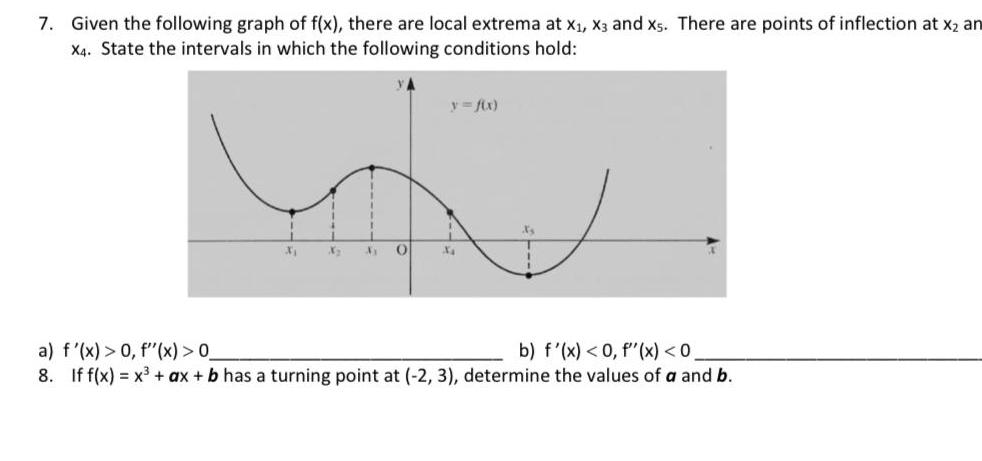Question:

# 7 Given the following graph of f x there are local extrema

Last updated: 11/17/20237 Given the following graph of f x there are local extrema at xX X3 and xs There are points of inflection at x an X4 State the intervals in which the following conditions hold X 4 X 31 O y f x X X 1 a f x 0 f x 0 b f x 0 f x 0 8 If f x x ax b has a turning point at 2 3 determine the values of a and b